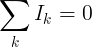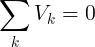# Kirchhoff's Laws

Kirchhoff's current law and voltage law, defined by Gustav Kirchhoff, describe the relation of values of currents that flow through a junction point and voltages in a an electrical circuit loop, in an electrical circuit.

## Kirchhoff's Current Law (KCL)

This is Kirchhoff's first law.

The sum of all currents that enter an electrical circuit junction is 0. The currents enter the junction have positive sign and the currents that leave the junction have a negative sign:Another way to look at this law is that the sum of currents that enter a junction is equal to the sum of currents that leave the junction:

#### KCL example

I1 and I2 enter the junction

I3 leave the junction

I1=2A, I2=3A, I3=-1A, I4= ?

Solution:

Ik = I1+I2+I3+I4 = 0

I4 = -I1 - I2 - I3 = -2A - 3A - (-1A) = -4A

Since I4 is negative, it leaves the junction.

## Kirchhoff's Voltage Law (KVL)

This is Kirchhoff's second law.

The sum of all voltages or potential differences in an electrical circuit loop is 0.#### KVL example

VS = 12V, VR1 = -4V, VR2 = -3V

VR3 = ?

Solution:

Vk = VS + VR1 + VR2 + VR3 = 0

VR3 = -VS - VR1 - VR2 = -12V+4V+3V = -5V

The voltage sign (+/-) is the direction of the potential difference.